## INDEX

(51) What will be output if you will compile and execute the following c code?

struct marks{
int p:3;
int c:3;
int m:2;
};
void main(){
struct marks s={2,-6,5};
printf("%d %d %d",s.p,s.c,s.m);
}

(a) 2 -6 5
(b) 2 -6 1
(c) 2 2 1
(d) Compiler error
(e) None of these

Explanation:
Binary value of 2: 00000010 (Select three two bit)
Binary value of 6: 00000110
Binary value of -6: 11111001+1=11111010
(Select last three bit)
Binary value of 5: 00000101 (Select last two bit)

Complete memory representation: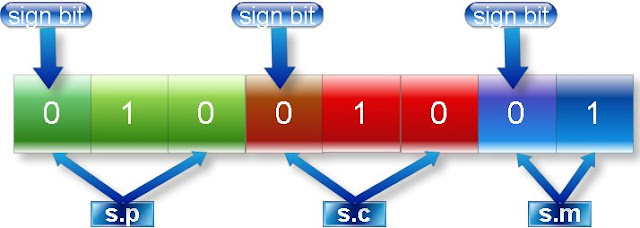(52) What will be output if you will compile and execute the following c code?

void main(){
static char *s={"math","phy","che"};
typedef char *( *ppp);
static ppp p1=&s,p2=&s,p3=&s;
char * (*(*array))={&p1,&p2,&p3};
char * (*(*(*ptr)))=&array;
p2+=1;
p3+=2;
printf("%s",(***ptr));

}

(a) math
(b) phy
(c) che
(d) Compiler error
(e) None of these

Explanation:
Here
ptr: is pointer to array of pointer to string.
P1, p2, p3: are pointers to array of string.
array: is array which contain pointer to array of string.
Pictorial representation: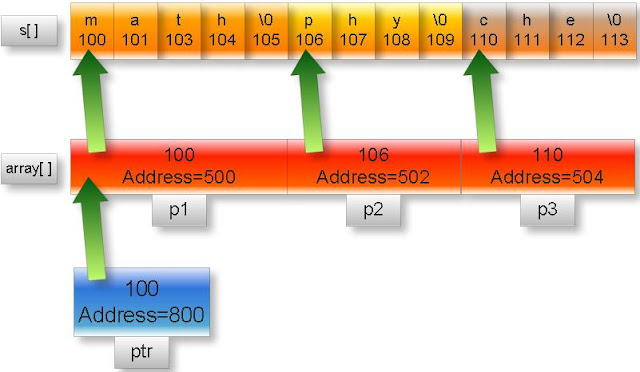Note: In the above figure upper part of box represent content and lower part represent memory address. We have assumed arbitrary address.

As we know p[i]=*(p+i)
(***ptr)=(*(***ptr+0))=(***ptr)
=(***(&array)) //ptr=&array
=(**array) //From rule *&p=p
=(**(&p1)) //array=&p1
=(*p1)
=(*&s) //p1=&s
=s=”che”

(53) What will be output if you will compile and execute the following c code?

#include"conio.h"
int display();
int(*array)();
int(*(*ptr))();
void main(){
array=display;
array=getch;
ptr=&array;
printf("%d",(**ptr)());
(*(*ptr+1))();
}
int display(){
int x=5;
return x++;
}

(a)5
(b)6
(c)0
(d)Compiler error
(e)None of these

Explanation:
In this example:
array []: It is array of pointer to such function which parameter is void and return type is int data type.

ptr: It is pointer to array which contents are pointer to such function which parameter is void and return type is int type data.

(**ptr)() = (** (&array)) () //ptr=&array
= (*array) () // from rule *&p=p
=array  () //from rule *(p+i)=p[i]
=display () //array=display

(*(*ptr+1))() =(*(*&array+1))() //ptr=&array
=*(array+1) () // from rule *&p=p
=array  () //from rule *(p+i)=p[i]
=getch () //array=getch

(54) What will be output if you will compile and execute the following c code?

void main(){
int i;
char far *ptr=(char *)0XB8000000;
*ptr='A';
*(ptr+1)=1;
*(ptr+2)='B';
*(ptr+3)=2;
*(ptr+4)='C';
*(ptr+5)=4;
}

It output will be A, B and C in blue, green and red color respectively. As shown in following figure: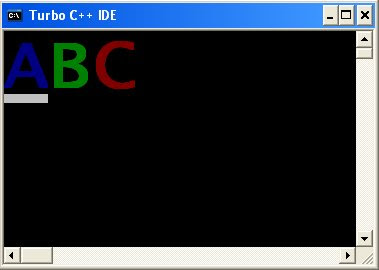(55) What will be output if you will compile and execute the following c code?

#include "dos.h"
void main(){
int j;
union REGS i,o;
char far *ptr=(char *)0XA0000000;
i.h.ah=0;
i.h.al=0x13;
int86(0x10,&i,&o);
for(j=1;j<=100;j++){
*(ptr+j)=4;
}

}

One red color line in the graphics console as shown in the following figure(56) What will be output if you will compile and execute the following c code?

void main(){
int huge*p=(int huge*)0XC0563331;
int huge*q=(int huge*)0xC2551341;
*p=200;
printf("%d",*q);
}

(a)0
(b)Garbage value
(c)null
(d) 200
(e)Compiler error

Explanation:

Physical address of huge pointer p

=0XC056 * 0X10 +0X3331
=0XC0560 + 0X3331
=0XC3891

Physical address of huge pointer q

=0XC255 * 0X10 +0X1341
=0XC2550 + 0X1341
=0XC3891

Since both huge pointers p and q are pointing same physical address so content of q will also same as content of q.

(57) Write c program which display mouse pointer and position of pointer.(In x coordinate, y coordinate)?

#include”dos.h”
#include”stdio.h”
void main()
{
union REGS i,o;
int x,y,k;
//show mouse pointer
i.x.ax=1;
int86(0x33,&i,&o);
while(!kbhit()) //its value will false when we hit key in the key board
{
i.x.ax=3; //get mouse position
x=o.x.cx;
y=o.x.dx;
clrscr();
printf("(%d , %d)",x,y);
delay(250);
int86(0x33,&i,&o);
}
getch();
}

(58) Write a c program to create dos command: dir.

Step 1: Write following code.

#include “stdio.h”
#include “dos.h”
void main(int count,char *argv[])
{
struct find_t q ;
int a;
if(count==1)
argv="*.*";
a = _dos_findfirst(argv,1,&q);
if(a==0)
{
while (!a)
{
printf(" %s\n", q.name);
a = _dos_findnext(&q);
}
}
else
{
}
}

Step 2: Save the as list.c (You can give any name)
Step 3: Compile and execute the file.
Step 4: Write click on My computer of Window XP operating system and select properties.
Step 5: Select Advanced -> Environment Variables
Step 6: You will find following window:
Click on new button (Button inside the red box)Step 7: Write following:
Variable name: path
Variable value: c:\tc\bin\list.c (Path where you have saved)Step 8: Open command prompt and write list and press enter.

Command line argument tutorial.

(59) What will be output if you will compile and execute the following c code?

void main(){
int i=10;
static int x=i;
if(x==i)
printf("Equal");
else if(x>i)
printf("Greater than");
else
printf("Less than");

}

(a) Equal
(b) Greater than
(c) Less than
(d) Compiler error
(e) None of above

Explanation:
static variables are load time entity while auto variables are run time entity. We can not initialize any load time variable by the run time variable.
In this example i is run time variable while x is load time variable.

(60) What will be output if you will compile and execute the following c code?
void main(){
int i;
float a=5.2;
char *ptr;
ptr=(char *)&a;
for(i=0;i<=3;i++)
printf("%d ",*ptr++);
}

(a)0 0 0 0
(b)Garbage Garbage Garbage Garbage
(c)102 56 -80 32
(d)102 102 -90 64
(e)Compiler error

Explanation:
In c float data type is four byte data type while char pointer ptr can point one byte of memory at a time.
Memory representation of float a=5.2ptr pointer will point first fourth byte then third byte then second byte then first byte.
Content of fourth byte:
Binary value=01100110
Decimal value= 64+32+4+2=102
Content of third byte:
Binary value=01100110
Decimal value=64+32+4+2=102
Content of second byte:
Binary value=10100110
Decimal value=-128+32+4+2=-90
Content of first byte:
Binary value=01000000
Decimal value=64

Note: Character pointer treats MSB bit of each byte i.e. left most bit of above figure as sign bit.

(61) What will be output if you will compile and execute the following c code?

void main(){
int i;
double a=5.2;
char *ptr;
ptr=(char *)&a;
for(i=0;i<=7;i++)
printf("%d ",*ptr++);
}

(a) -51 -52 -52 -52 -52 -52 20 64
(b) 51 52 52 52 52 52 20 64
(c) Eight garbage values.
(d) Compiler error
(e) None of these

Explanation:
In c double data type is eight byte data type while char pointer ptr can point one byte of memory at a time.
Memory representation of double a=5.2ptr pointer will point first eighth byte then seventh byte then sixth byte then fifth byte then fourth byte then third byte then second byte then first byte as shown in above figure.

Content of eighth byte:
Binary value=11001101
Decimal value= -128+64+8+4+1=-51
Content of seventh byte:
Binary value=11001100
Decimal value= -128+64+8+4=-52
Content of sixth byte:
Binary value=11001100
Decimal value= -128+64+8+4=-52
Content of fifth byte:
Binary value=11001100
Decimal value= -128+64+8+4=-52
Content of fourth byte:
Binary value=11001100
Decimal value= -128+64+8+4=-52
Content of third byte:
Binary value=11001100
Decimal value= -128+64+8+4=-52
Content of second byte:
Binary value=000010100
Decimal value=16+4=20
Content of first byte:
Binary value=01000000
Decimal value=64

Note: Character pointer treats MSB bit of each byte i.e. left most bit of above figure as sign bit.

(62) What will be output if you will compile and execute the following c code?

void main(){
printf("%s","c" "question" "bank");
}

(a) c question bank
(b) c
(c) bank
(d) cquestionbank
(e) Compiler error

Explanation:
In c string constant “xy” is same as “x” “y”

(63) What will be output if you will compile and execute the following c code?

void main(){
printf("%s",__DATE__);
}

(a) Current system date
(b) Current system date with time
(c) null
(d) Compiler error
(e) None of these

Explanation:
__DATE__ is global identifier which returns current system date.

(64) What will be output if you will compile and execute the following c code?

void main(){
char *str="c-pointer";
printf("%*.*s",10,7,str);
}

(a) c-pointer
(b) c-pointer
(c) c-point
(d) cpointer null null
(e) c-point

Explanation:
Meaning of %*.*s in the printf function:
First * indicates the width i.e. how many spaces will take to print the string and second * indicates how many characters will print of any string.

Following figure illustrates output of above code: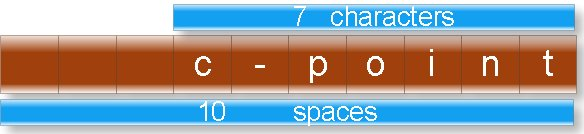(65) What will be output if you will compile and execute the following c code?

void start();
void end();
#pragma startup start
#pragma exit end
int static i;
void main(){
printf("\nmain function: %d",++i);
}
void start(){
clrscr();
printf("\nstart function: %d",++i);
}
void end(){
printf("\nend function: %d",++i);
getch();
}

(a)
main function: 2
start function: 1
end function:3

(b)
start function: 1
main function: 2
end function:3

(c)
main function: 2
end function:3
start function: 1

(d) Compiler error
(e) None of these

Explanation:
Every c program start with main function and terminate with null statement. But #pragma startup can call function just before main function and #pragma exit

(66) What will be output if you will compile and execute the following c code?

void main(){
int a=-12;
a=a>>3;
printf("%d",a);
}

(a) -4
(b) -3
(c) -2
(d) -96
(e) Compiler error

Explanation:

Binary value of 12 is: 00000000 00001100
Binary value of -12 wills 2’s complement of 12 i.e.So binary value of -12 is: 11111111 11110100Right shifting rule:
Rule 1: If number is positive the fill vacant spaces in the left side by 0.
Rule 2: If number is negative the fill vacant spaces in the left side by 1.
In this case number is negative. So right shift all the binary digits by three space and fill vacant space by 1 as shown following figure:Since it is negative number so output will also a negative number but its 2’s complement.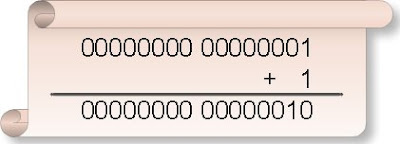Hence final out put will be:And its decimal value is: 2
Hence output will be:-2

(67) What will be output if you will compile and execute the following c code?

#include "string.h"
void main(){
clrscr();
printf("%d %d",sizeof("string"),strlen("string"));
getch();
}

(a) 6 6
(b) 7 7
(c) 6 7
(d) 7 6
(e) None of these

Explanation:
Sizeof operator returns the size of string including null character while strlen function returns length of a string excluding null character.

(68) What will be output if you will compile and execute the following c code?

void main(){
static main;
int x;
x=call(main);
clrscr();
printf("%d ",x);
getch();
}
}

(a) 0
(b) 1
(c) Garbage value
(d) Compiler error
(e) None of these

Explanation:
As we know main is not keyword of c but is special type of function. Word main can be name variable in the main and other functions.

(69) What will be output if you will compile and execute the following c code?

void main(){
int a,b;
a=1,3,15;
b=(2,4,6);
clrscr();
printf("%d ",a+b);
getch();
}

(a) 3
(b) 21
(c) 17
(d) 7
(e) Compiler error

Explanation:
In c comma behaves as separator as well as operator.
a=1, 3, 15;
b= (2, 4, 6);
In the above two statements comma is working as operator. Comma enjoys least precedence and associative is left to right.

Assigning the priority of each operator in the first statement: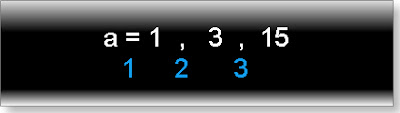Hence 1 will assign to a.
Assigning the priority of each operator in the second statement: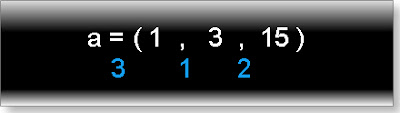(70) What will be output if you will compile and execute the following c code?

int dynamic(int,...);
void main(){
int x,y;
x=dynamic(2,4,6,8,10,12,14);
y=dynamic(3,6,9,12);
clrscr();
printf("%d %d ",x,y);
getch();
}
int dynamic(int s,...){
void *ptr;
ptr=...;
(int *)ptr+=2;
s=*(int *)ptr;
return s;

}

(a) 8 12
(b) 14 12
(c) 2 3
(d) Compiler error
(e) None of these

Explanation:
In c three continuous dots is known as ellipsis which is variable number of arguments of function. In this example ptr is generic pointer which is pointing to first element of variable number of argument. After incrementing it will point third element.

(71) What will be output if you will compile and execute the following c code?

int extern x;
void main()
printf("%d",x);
x=2;
getch();
}
int x=23;

(a) 0
(b) 2
(c) 23
(d) Compiler error
(e) None of these

Explanation:
extern variables can search the declaration of variable any where in the program.

(72) What will be output if you will compile and execute the following c code?

void main(){
int i=0;
if(i==0){
i=((5,(i=3)),i=1);
printf("%d",i);
}
else
printf("equal");
}

(a) 5
(b) 3
(c) 1
(d) equal
(e) None of above

Explanation:

(73) What will be output if you will compile and execute the following c code?

void main(){
int a=25;
clrscr();
printf("%o %x",a,a);
getch();
}

(a) 25 25
(b) 025 0x25
(c) 12 42
(d) 31 19
(e) None of these

Explanation:
%o is used to print the number in octal number format.
%x is used to print the number in hexadecimal number format.

Note: In c octal number starts with 0 and hexadecimal number starts with 0x.

(74) What will be output if you will compile and execute the following c code?

#define message "union is\
power of c"
void main(){
clrscr();
printf("%s",message);
getch();
}

(a) union is power of c
(b) union ispower of c
(c) union is
Power of c
(d) Compiler error
(e) None of these

Explanation:
If you want to write macro constant in new line the end with the character \.

(75) What will be output if you will compile and execute the following c code?

#define call(x) #x
void main(){
printf("%s",call(c/c++));
}

(a)c
(b)c++
(c)#c/c++
(d)c/c++
(e)Compiler error

Explanation:
# is string operator. It converts the macro function call argument in the string. First see the intermediate file:

test.c 1:
test.c 2: void main(){
test.c 3: printf("%s","c/c++");
test.c 4: }
test.c 5:

It is clear macro call is replaced by its argument in the string format.

(75) What will be output if you will compile and execute the following c code?

void main(){
if(printf("cquestionbank"))
printf("I know c");
else
printf("I know c++");
}

(a) I know c
(b) I know c++
(c) cquestionbankI know c
(d) cquestionbankI know c++
(e) Compiler error